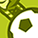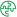App Development

Announcements
QlikWorld 2022, LIVE in Denver CO., May 16-19, 2022. REGISTER NOW TO RECEIVE EARLY BIRD PRICING
cancel
Showing results for
Did you mean:Contributor II

[SOLVED] Dynamic Filter Bookmark Unique Selection

Hi All,

I am trying to automatically filter when user log in the last Cycle which should be the max value of the field.

This is a single selection filter field.

Field = [Cycle]

Values of Field = {"Actual, 92020, 102020, 122020, 42021, 52021"}  // date as "myyyy" format.

For the values above, the selected should be 52021.

How can I achieve this and save as dynamic bookmark.

1 Solution

Accepted SolutionsContributor II
Author

Few months later... I'VE JUST SOLVED!

I was facing problems by using dynamic filter selections, because some of my calculated measures was using "GetFieldSelection". Thus, since my selection was now given by the formula below, not the value, it couldn't be calculated.

=only({1}Cycle)=FieldValue('Cycle',FieldValueCount('Cycle'))

In order to solve, instead of using "GetFieldSelection", I changed to max of field (since it was an unique selection).

Also I changed a lit bit the formula to get the max values no matter the months 12022 and 122021 (12022 should be the max).

Final Filter Selection

=only({1}Cycle)= \$(vMaxCycle)

where vMaxCycle is given by:

=Num(
concat(right(Max({1}TOTAL RIGHT(Cycle,4)&if(len(Cycle)=5,'0'&left(Cycle,1),left(Cycle,2))),2)
&
left(Max({1}TOTAL RIGHT(Cycle,4)&if(len(Cycle)=5,'0'&left(Cycle,1),left(Cycle,2))),4),'')
)

3 RepliesPartner

Hi,
assuming you always want the last record that was added to the field.
you should paste this expression to the field search box
and then create a bookmark

=Cycle=fieldvalue('Cycle',FieldValueCount('Cycle'))Contributor II
Author

It couldn't find any match, but I think it was due set analysis.

When I included only and {1} to ignore the selections, I was able to get the right value, but when saving the bookmark it doesn't save as formula but already transformed to the value.

=only({1}Cycle)=FieldValue('Cycle',FieldValueCount('Cycle'))

I already tried disabling the unique selection, saving the bookmark and afterwards making it unique again, but no success.Contributor II
Author

Few months later... I'VE JUST SOLVED!

I was facing problems by using dynamic filter selections, because some of my calculated measures was using "GetFieldSelection". Thus, since my selection was now given by the formula below, not the value, it couldn't be calculated.

=only({1}Cycle)=FieldValue('Cycle',FieldValueCount('Cycle'))

In order to solve, instead of using "GetFieldSelection", I changed to max of field (since it was an unique selection).

Also I changed a lit bit the formula to get the max values no matter the months 12022 and 122021 (12022 should be the max).

Final Filter Selection

=only({1}Cycle)= \$(vMaxCycle)

where vMaxCycle is given by:

=Num(
concat(right(Max({1}TOTAL RIGHT(Cycle,4)&if(len(Cycle)=5,'0'&left(Cycle,1),left(Cycle,2))),2)
&
left(Max({1}TOTAL RIGHT(Cycle,4)&if(len(Cycle)=5,'0'&left(Cycle,1),left(Cycle,2))),4),'')
)Tags
Community Browser